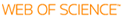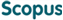### BROWSE

#### Related ResearcherKim, Jaeup U.
Nanostructured Polymer Materials Theory Lab
Research Interests
• block copolymers, soft matter, nanostructures & patterning, machine learning

Finite-N effects for ideal polymer chains near a flat impenetrable wall

Cited 8 times inCited 7 times inTitle
Finite-N effects for ideal polymer chains near a flat impenetrable wall
Author
Keywords
05.70.Np Interface and surface thermodynamics; 61.25.he Polymer solutions; 82.35.Gh Polymers on surfaces adhesion; Dependent functions; Diffusion equations; Dirichlet boundary condition; Gaussian corrections; Hard walls; Impenetrable walls; Monomer density; Partition functions; Polymer chains; Positive coefficients; Quantity of interest; Robin boundary conditions
Issue Date
2009-05
Publisher
SPRINGER
Citation
EUROPEAN PHYSICAL JOURNAL E, v.29, no.1, pp.107 - 115
Abstract
This paper addresses the statistical mechanics of ideal polymer chains next to a hard wall. The principal quantity of interest, from which all monomer densities can be calculated, is the partition function, G(N)(z), for a chain of N discrete monomers with one end fixed a distance z from the wall. It is well accepted that in the limit of infinite N, G(N)(z) satisfies the diffusion equation with the Dirichlet boundary condition, G(N)(0) = 0, unless the wall possesses a sufficient attraction, in which case the Robin boundary condition, G(N)(0) = -xi G'(N)(0), applies with a positive coefficient, xi. Here we investigate the leading N(-1/2) correction, G(N)(z). Prior to the adsorption threshold, Delta G(N)(z) is found to involve two distinct parts: a Gaussian correction (for z less than or similar to aN(1/2)) with a model-dependent amplitude, A, and a proximal-layer correction (for z less than or similar to a) described by a model-dependent function, B(z).
URI
DOI
10.1140/epje/i2009-10454-2
ISSN
1292-8941
Appears in Collections:
SNS_Journal Papers
Files in This Item: